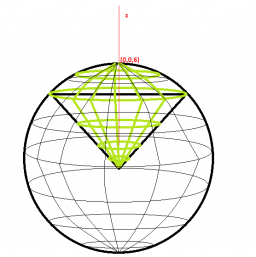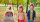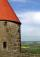# The rotating

The rotating cone has a height of 0.9 m and the diameter of the base is 7.2 dm. Calculate the surface of the cone. (Hint: use Pythagorean theorem for a side of cone)

S =  150.3436 dm2

### Step-by-step explanation:Did you find an error or inaccuracy? Feel free to write us. Thank you!Tips to related online calculators
Pythagorean theorem is the base for the right triangle calculator.

#### You need to know the following knowledge to solve this word math problem:

We encourage you to watch this tutorial video on this math problem:

## Related math problems and questions:

• Rotating coneCalculate the volume and the surface area of a rotating cone of base radius r = 2.3 dm and a height h = 46 mm.
• The volumeThe volume of the cone is 94.2dm³, the radius of the base is 6 dm Calculate the surface of the cone.
• Cone area and sideCalculate the surface area and volume of a rotating cone with a height of 1.25 dm and 17,8dm side.
• The Indian tentThe Indian tent is cone-shaped. Its height is 3.5 m. The diameter of the base is 2.5 m. How much canvas is needed to make a tire?
• ConeCalculate volume and surface area of ​​the cone with a diameter of the base d=15 cm and side of the cone with the base has angle 52°.
• Surface and volumeFind the surface and volume of the rotating cone if the circumference of its base is 62.8 m and the side is 25 m long.
• Cone - from volume surface areaThe volume of the rotating cone is 1,018.87 dm3, and its height is 120 cm. What is the surface area of the cone?
• Hexagonal pyramidRegular hexagonal pyramid has dimensions: length edge of the base a = 1.8 dm and the height of the pyramid = 2.4 dm. Calculate the surface area and volume of a pyramid.The quadrilateral pyramid has a rectangular base of 24 cm x 3.2dm and a body height of 0.4m. Calculate its volume and surface area.
• Rotating cone IICalculate area of surface of rotating cone with base radius r=19 cm and height h=9 cm.
• Prism - boxThe base of prism is a rectangle with a side of 7.5 cm and 12.5 cm diagonal. The volume of the prism is V = 0.9 dm3. Calculate the surface of the prism.
• PrismFind the volume and surface area of prism with base of an equilateral triangle with side 7 dm long and the body height of 1.5 m.
• The pyramid 4sThe pyramid with a rectangular base measuring 6 dm and 8 dm has a side edge of length 13 dm. Calculate the surface area and volume of this pyramid.
• Rotating coneFind the rotating cone's surface and volume if its side is 150 mm long and the circumference of the base is 43.96 cm.
• Volume of the coneFind the volume of the cone with the base radius r and the height v. a) r = 6 cm, v = 8 cm b) r = 0,9 m, v = 2,3 m c) r = 1,4 dm, v = 30 dm
• Cone and the ratioThe rotational cone has a height 43 cm, and the ratio of the base surface to lateral surface is 5: 7. Calculate the surface of the base and the lateral surface.
• The roof of the churchThe cone roof of the church has a diameter of 3 m and a height of 4 m. What is the size of the side edge of the church roof (s=?) and many sheets sheet will be needed to cover the church roof?